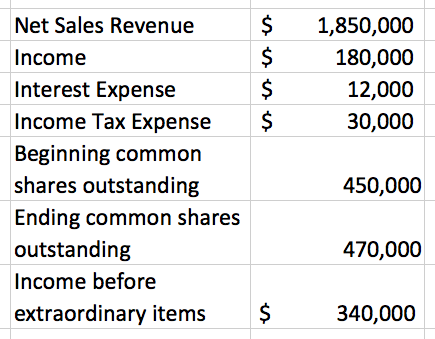# Problem: Consider the following information: Compute the earnings per share to the nearest cent. A. $0.48 B.$0.39 C. $0.25 D.$0.74

###### Problem Details

Consider the following information:

Compute the earnings per share to the nearest cent.

A. $0.48 B.$0.39

C. $0.25 D.$0.74